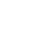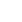# Exchanging Time

JRDAssist
Middle School and High School Teacher

The lesson is used for students to practice basic time measurement, and understanding the basic units of time.

##Length of Time: 2-3 Class Periods

### Common Core Alignment

CCSS.Math.Content.3.MD.A.1 - Tell and write time to the nearest minute and measure time intervals in minutes. Solve word problems involving addition and subtraction of time intervals in minutes, e.g., by representing the problem on a number line diagram.

## Objectives & Outcomes

The students will be able to use the four operations to convert units of time, and measure the passage of time, using seconds, minutes, hours, days, months, and years.

## Materials Needed

paper plates, brads, scissors, paper punch, markers

Prepare ahead of time:  Time conversion chart for each student, gather supplies as needed for clock making, adapt clock making as necessary.

## Procedure

### Body of Lesson

#### Modeling

• Display actual time measurement tools (clocks, calendars, stopwatch, etc.) or the images of the same.
• Ask students why each measurement tool is used.  What is it used for?  (Measuring days, hours, minutes, seconds – races, meetings, for cooking, etc.)
• Allow for responses and discussion.
• Tell students they will need to learn the units of time, and how to measure time.

#### Guided Practice

• Give students a chart showing the different units of time and conversions.  (60 sec. = 1 min, 60 min = 1 hour, etc.)
• Distribute clocks with movable hands.  (These can be made easily using cardboard or paper plates: http://lifestyle.howstuffworks.com/crafts/paper-crafts/paper-clocks.htm)
• Allow time for students to build their paper plate clocks if necessary.
• Review each unit of time with students:  seconds, minutes, hours, days (optional: months/years, decades, centuries)
• Using the clocks, show students the passage of time in minutes and hours.  Give students examples of the passage of time:  How many minutes/hours have passed between 10:00 and 10:50, 12:45 to 1:55, etc.?  How many minutes’ old are they?
• Use as many examples as necessary based on students’ needs.
• Tell students they will now create ten problems, which will be solved by another student.  Tell students to mix up the questions and to use real life events.  (For example, how much time passes during the school day from 8:30 AM to 3:15 PM, days in a school year, etc.  How many seconds long is gym class?)
• Give students time to complete their problems.  Tell them to create an Answer Key.
• Once finished, distribute the problems randomly to other students.  Give students time to answer each problem.
• Once completed, they will exchange the problems to be checked by the original writer.

#### Independent Practice

• Create worksheets related to the passage of time and time unit conversion.

### Closing

• Ask students: Why do you think time goes faster when you are having fun, but seems to slow down when you are doing something that is not fun?
• Allow for responses and discussion.

## Assessment & Evaluation

Create a quiz or test with time problems.  Collect student-created problems to be assessed.

## Modification & Differentiation

Use teacher-created worksheets or resources from the Internet for additional student practice.  Allow students to work in pairs.  Give students ample practice with their clocks, verbally giving times to show on the clock, passage of time, etc.  Use Interactive Internet resources if students have access to computers.  Use digital clocks as well.  Make sample digital clocks.

## Related Lesson Plans

##### Shape Up

This lesson will allow students to demonstrate knowledge of various grade appropriate shapes.

##### The Value of a Number

The students will work in groups of 4-6 physically learning and reviewing place value.

##### Comparing Multiplication Facts (Hey Tocayo!)

Students will be assigned a number that has various factors and they will find partners with different factors that have the same product.

##### Graphing With Insects

This lesson is designed to teach students to draw a picture graph and a bar graph (with single-unit scale) to represent a data set with up to four categories. Plus, solve simple put-together, take-apart, and compare problems.

##